Tamilnadu State Board New Syllabus Samacheer Kalvi 8th Maths Guide Pdf Chapter 7 Information processing Ex 7.3 Text Book Back Questions and Answers, Notes.

## Tamilnadu Samacheer Kalvi 8th Maths Solutions Chapter 7 Information processing Ex 7.3

Question 1.
Fill in the blanks (Use Atbash Cipher that is given in code 3)
Hint:
For this question, we need to use Atbash cipher. For Atbash cipher, first we write the alphabets from A to Z and then in reverse from Z to A below that.
A B C D E F G H I J K L M N O P Q R S T U V W X Y Z
Z Y X W V U T S R Q P O N M L K J I H G F E D C B A(i) G Z N R O = _________
TAMIL
Hint:
Now to solve, we look up the corresponding letter from the table to replace in code to get the actual word.
So, for G Z N R O, from table,
for G, it is T
for Z, it i sA
for N, it is M
for R, it is i
for 0, it is L
So, the actual word is TAMIL

(ii) V M T O R H S = ________
ENGLISH

Hint:
VMTORHS
To solve, we look up the corresponding letter from table to replace in code to get the actual word.
For V, it is E
for M, it is N
for T, it is G
for O, it is L
for R, it is I,
for H, it is S
for S, it is H
Therefore we get E N G L I S H = ENGLISH(iii) N Z G S V N Z G R X H _________
MATHEMATICS
Hint:
Similarly as above for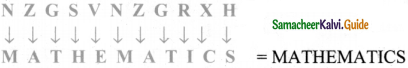(iv) H X R V M X V __________
SCIENCE
Hint:
For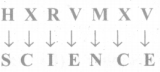(v) H L X R Z O H X R V M X V = __________
SOCIAL SCIENCE
Hint: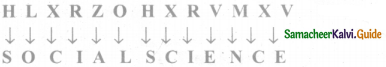Question 2.
Match the following (a = 00 ………… Z = 25).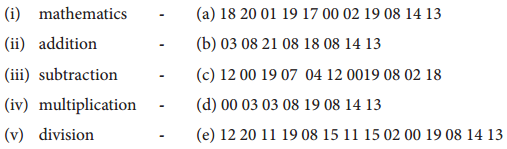(i) – c
(ii) – d
(iii) – a
(iv) – e
(v) – b
Hint:
(i) Mathematics is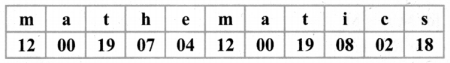So matching option is c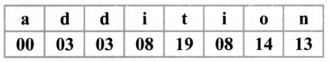Matching option is d(iii) Subtraction is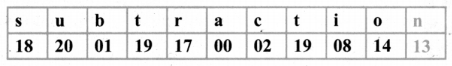multiplication is a

(iv) multiplication is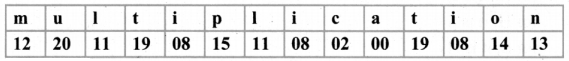Matching option is e
however instead of 25, it should be 08

(v) division is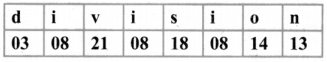Matching option is b

Question 3.
Frame Additive cipher table (key = 4).
Step 1 : write all alphabets
Step 2 : Assign numbers to each alphabet starting from 00 till 25.
Step 3 : add key value (here it is 4) to the numbers assigned in step 2 to form cipher table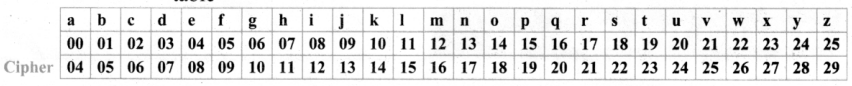Question 4.
A message like “Good Morning” written in reverse would instead be “Doog Gninrom” In the same way decode the sentence given below:
“Ot dnatsrednu taht scitamehtam nac eb decneirepxe erehwreve ni erutan dna laer efil”
Given that good morning written in reverse is doog gninrom.
We have to decode the below by reversing, so,
Ot dnatsrednu taht scitamehtam nac eh decneirepxe erehwreve ni erutan dna laer efil.

Ans: to understand that mathematics can be experienced everywhere in nature and real life.

Question 5.
Decode the given Pigpen Cipher text and compare your answer to get result.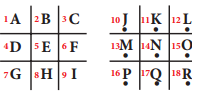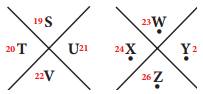I. The room number in which the treasure took place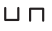The room in which the treasure took place = 28

II. Place of the treasure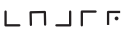The place of treasure = Chair

III. The name of the treasure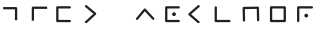Identity of treasure = Gift voucher.Question 6.
Praveen recently got the registration number for his new two-wheeler. Here, the number is given in the form of mirror-image. Encode the image and find the correct registration number of praveen’s two-wheeler.
T N 1 2 H 2 5 8 9
(a)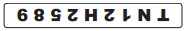(b)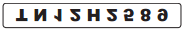(c)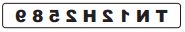(d)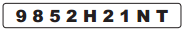The mirror image isWhen we place an imaginary mirror & visualize the image seen in the mirror, we will get the below.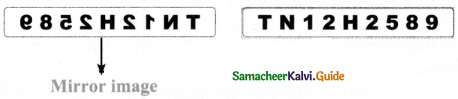Objective Type Questions

Question 7.
In questions (i) and (ii), there are four groups of letters in each set. Three of these sets are alike in some way while one is different. Find the one which is different.
(i).(A) C R D T
(B) A P B Q
(C) E U F V
(D) G W H X
(i) (A) C R D T
Hint:
The four groups of letters are
CRDT APBQ EUFV GWHX
The above can be written as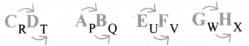We find that when we take 1st & 3rd letter & 2nd & 4th letter as 2 pairs, the 3 letter is the next letter alphabetically to the 1st letter.
Similarly the 4th letter is alphabetically the next letter of the 2nd letter.
i.e CD, AB, EF, GH & PQ, UV, WX
Only in CRDT, we have T instead of ‘S’
So, Ans: in CRDT ⇒ Option (a)(ii). (A) H K N Q
(B) I L O R
(C) J M P S
(D) A D G J
(D) A D G J
Hint:
The four groups of letters are
If we notice, we find that 2 letters are missing in the sequence. le.
HIJ KLI NOP Q
IJK LMN OPQ R
JKL MNO PQR S
ABC DEF GH I
We find that only in ADGI, the difference is only one letter between G & I.
Hence it is the odd one out.Question 8.
A group of letters are given. A numerical code has been given to each letter. These letters have to be unscrambled into a meaningful word. Find out the code for the word so formed from the 4 answers given.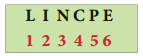(A) 2 3 4 1 5 6
(B) 5 6 3 4 2 1
(C) 6 1 3 5 2 4
(D) 4 2 1 3 5 6
(B) 5 6 3 4 2 1

Hint:
Given code isOption (a) is 234156. When we substitute number for each letter from code, we get,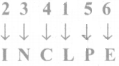Option (b) is 563421, similarly, we get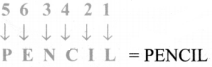Option (c) is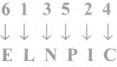Option d) is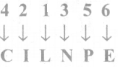So, only in option b, we get a meaningful word, i.e PENCIL.Question 9.
Questions (iii) and (iv) are based on code language. Find the correct answer from the four alternatives given.
(iii)Inacertain code, ‘M E D I C I N E’ is coded as ‘E O J D J E F M’,then how is ‘C O M P U T E R’ written in the same code?
(A) C N P R V U F Q
(B) C M N Q T U D R
(C) R F I J V Q N P C
(D) R N V F T U D Q
(C) R F I J V Q N P C
Hint:
lt is given that in a certain code MEDlClNE is coded as E O J D J E F M
When we observe the word & the code, we find that, there is a pattern.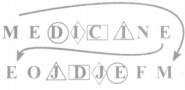[to understand, see the matching shapes]
To get the code from the word, we follow the below steps

1.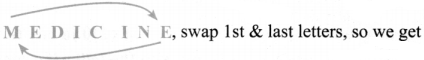E [E D I C I N] M

2. For the middle letters, replace the letters with their alphabetically next letters, so we get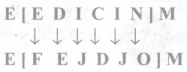3. Now we have to reverse the order of the middle letters in the bracket, so we get
E [O J D J E F] M = E O J D J E F M
Thus we get the code
So similarly, we have to follow the 3 steps to get code, therefore: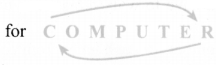Step 1: Swap 1st & last letters, so we get
R [O M P U T E] C

Step 2: For the middle letters, replace the letters with alphabetically next letters, so we get
R [P N Q V L F] C

Step 3: Reverse the word of letters in the bracket to we get
R [F U V Q N P] C
∴ Ans is R F U V Q N P C ⇒ option c(iv) lf the word ’P H O N E’ is coded as ’S K R Q H’, how will ’R A D I O’ be coded?
(A) S C G N H
(B) V R G N G
(C) U D G L R
(D) S D H K Q
(C) U D G L R
Hint:
If PHONE is coded as S K R Q H
Find that code for R A D I O

We find that we get the code, by 3 letter alphabetically so. from P. skipping Q & R. we get S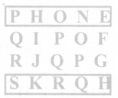Similarly, from H, skipping I & J, we get K
Like wise for R A D I O. skipping the 2 alphabets,
From R skip S & T → U
From A skip B & C → D
From D skip E & F → G
From l skip J & K → L
From O skip P & Q → R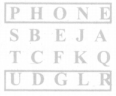∴ Ans is UDGLR ⇒ option c